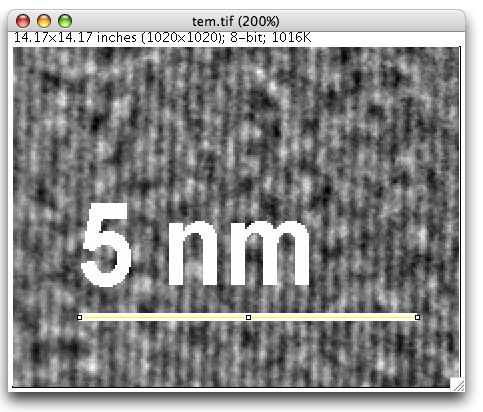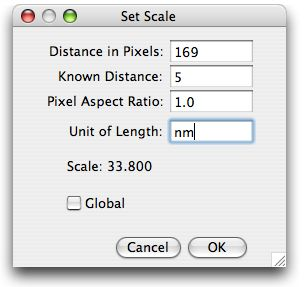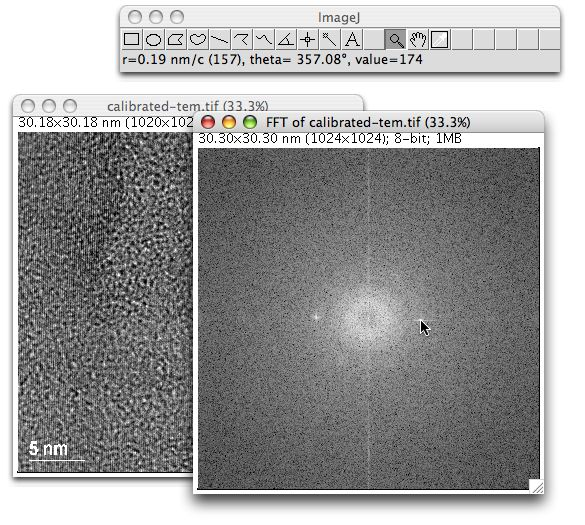FFT Measurements

This example demonstrates how to use FFTs to measure distances between atomic planes in high resolution transmission electron microscopy (TEM) images. It requires ImageJ 1.33i or later.Figure 1. High resolution TEM image.

The atomic planes are in the upper left corner. The image scale is provided by the bar (in nanometers) in the lower left corner. The orginal 1020x1020 TIFF image is also available.Figure 2. Scale bar with with straight line selection.

To spatially calibrate the image, zoom in on the scale bar, select it with the straight line selection tool, then select Analalyze/Set Scale.Figure 3. Set Scale dialog.

In the Set Scale dialog, set "Known Distance" to 5, "Unit of Length" to "nm", then click "OK". Note how the length of the scale bar was automatically entered into the "Distance in Pixels" field.Figure 5. Calibrated TEM image and FFT.

To measure the spacing of the atomic planes, use Process/FFT to calculate the FFT, move the cursor to the point in the FFT that represents the planes, and the spacing of the planes (0.19nm/cycle) will be displayed in ImageJ's status bar.Figure 6. Profile plot of atomic planes.

Another way to measure the spacing of the atomic planes is to generate a profile plot, manually count the cycles, then divide the distance (in this case 3.61) by the number of cycles (19).

TEM image contributed by Rodrigo Andrade, Federal University of Minas Gerais, Belo Horizonte, Brasil.

| Examples | Home |